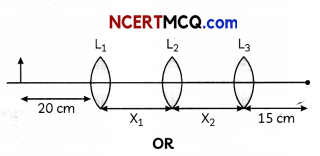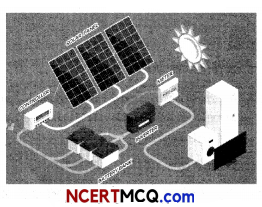Students can access the CBSE Sample Papers for Class 12 Physics with Solutions and marking scheme Term 2 Set 11 will help students in understanding the difficulty level of the exam.

## CBSE Sample Papers for Class 12 Physics Standard Term 2 Set 11 for Practice

Time Allowed: 2 Hours
Maximum Marks: 40

General Instructions:

• There are 12 questions in all. All questions are compulsory.
• This question paper has three sections: Section A, Section B and Section C.
• Section A contains three questions of two marks each, Section B contains eight questions of three marks each, Section C contains one case study-based question of five marks.
• There is no overall choice. However, an internal choice has been provided in one question of two marks and two questions of three marks. You have to attempt only one of the chokes in such questions.
• You may use log tables if necessary but use of calculator is not allowed.

SECTION – A
(Section A contains 3 questions of 2 marks each.)

Question 1.
Define the following terms:
(A) V-l characteristic of a forward biased diode.
(B) Reverse breakdown voltage. (2)

Question 2.
Which of the following is more stable and why ($${ }_{7}^{3} X$$) and ($${ }_{3}^{4} Y$$)?
OR
How the width of depletion layer changes in p-n junction diode when the junction is forward biased and reverse biased? (2)Question 3.
(A) Distinguish between an astronomical telescope and a terrestrial telescope?
(B) Find the magnification at least distance of distinct vision of a simple microscope having focal length 5 cm? (2)

SECTION – B
(Section B consists of 8 questions of 3 marks each.)

Question 4.
(A) In Young’s double slit experiment, what is the shape of interference fringes?
(B) The following table gives data about the single slit diffraction experiment:

 Wavelength of light Half angular width of the principal maxima λ θ Pλ Qθ

Find the ratio of the widths of the slits used in the two cases. Would the ratio of the half angular widths of the first secondary maxima, in the two cases, be also equal to q? (3)

Question 5.
A deuteron and an alpha particle are accelerated with the same accelerating potential.
Which one of the two has:
(i) greater value of de-Broglie wavelength, associated with it, and
(ii) less kinetic energy ? Explain. (3)
OR
(A) Work function of aluminium is 4.2 eV. If two photons, each of energy 2.5 eV, are incident on its surface, will the emission of electrons take place? Justify your answer.
(B) Work function of caesium and platinum are 2.14 eV and 5.65 eV, respectively.
Which one of the two metals has higher threshold wavelength? Justify. (3)

Question 6.
How does a microwave oven work on the principle of EM waves? (3)

Question 7.
A 12.5 eV electron beam is used to bombard gaseous hydrogen at room temperature. Up to which energy level the hydrogen atoms would be excited? Calculate the wavelength of the first member of Lyman and first member of Balmer series. (3)Question 8.
Find out the ratio of fringe width in Young’s double slit experiment when wavelength becomes four times. (3)

Question 9.
You are given three lenes L( L2, L3 each of focal length 15 cm. An object is kept at 20 cm in front of L1, as shown. The final real image is formed at the focus T of L3. Find the separations between L1 L2 and L3.Plot a graph to show variation of the angle of deviation as a function of angle of incidence for light passing through a prism. Derive an expression for refractive index of the prism in terms of angle of minimum deviation and angle of prism. (3)

Question 10.
Draw a labelled diagram of experimental setup of Rutherford’s alpha particle scattering experiment and write two important inferences drawn from the experiment. (3)

Question 11.
Define wavefront. Use Huygens’ principle to verify the laws of refraction. (3)SECTION – C
(Section C consists one case study-based question of 5 marks.)

Question 12.
As the world is advancing towards sustainable energy, solar cells play a crucial role for attaining that goal for the world. Solar panels used in industries, offices and homes are nothing but layers of conductors made of silicon to convert solar energy into electrical energy. As radiation strike the electron in the solar cell, the electron energy to jump from valence band to conduction band. This causes a motion of charge which generates electric current. Due to spontaneous reaction of the conversion, we can generate electrical energy during the daytime and store it in batteries which can be used in dark. The efficiency of semiconductors makes them a reliant source.Based on the above facts, answer the following questions:

(A) What should be the band gap of a material for it to be used as a solar cell?
(i) 1-1.8 eV
(ii) 0.5-1.3 eV
(iii) 1-2 eV
(iv) None of the above

(B) A solar cell is a:
(i) p-n junction
(ii) n-type semiconductor
(iii) p-type semiconductor
(iv) Intrinsic semiconductor

(C) What is the difference between photodiode and solar cell?
(i) No external bias in photodiode
(ii) No external bias in solar cell
(iii) Larger surface area in photodiode
(iv) No difference(D) Which of the following should not be the characteristic of a solar cell material?
(i) High absorption
(ii) High conductivity
(iii) High energy band
(iv) High availability

(E) Which of the following cannot be used as solar cells?
(i) Si
(ii) GaAs
(iii) CdS
(iv) PbS (5)